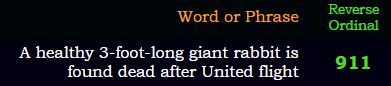## Cipher## Apr 26, 2017

### CNN - 3 foot long giant rabbit died on United Airlines flight?

http://edition.cnn.com/2017/04/26/travel/bunny-death-united-trnd/index.html
Earlier today I was checking CNN and I noted that they published a story on Dr. David Dao once again, so I was wondering why they were bringing it up today and this seems to be the reason, today they are talking about a giant 3 foot rabbit that died after United Airlines flight, this has to be at least 3rd or 4th time that something is happening on United Airlines flight in the last 2 months and it's really getting hilarious at this pointYou know it's a really good story when they open with 911 coded into the headlineUnited Airlines planes were supposedly used for the 9/11 attackThe rabbit was flying from "Heathrow Airport to O'Hare" = 1+4+8+7+1+9+3+4 + 8+9+9+2+3+9+7 + 7+3 + 3 + 1+8+9+4 = 119 (Reverse Reduction)
three foot long giant rabbit = 2+8+9+5+5 + 6+6+6+2 + 3+6+5+7 + 7+9+1+5+2 + 9+1+2+2+9+2 = 119 (Full Reduction)

Rabbit Simon died on April 19th on a date with 33 numerology (4 + 19 +2+0+1+7 = 33) and the story is coming 7 days later- one week = 6+5+5 + 5+5+5+2 = 33 (Full Reduction)"seven days" = 1+5+4+5+5 + 4+1+7+1 = 33 (Full Reduction)Darius = 23+26+9+18+6+8 = 90 (Reverse Ordinal)
Ninety = 5+9+5+5+2+7 = 33 (Full Reduction)April = 8+2+9+9+6 = 34 (Reverse Reduction)
Rabbit = 90+1+2+2+9+200 = 304 (English Extended)
Simon = 10+9+4+6+5 = 34 (Single Reduction)Note how their emphasize "Saddened" = 8+8+5+5+4+4+4+5 = 43 (Reverse Reduction)
Also "saddened" and Simon have matching gematria
"Simon" = 8+9+5+3+4 = 29 (Reverse Reduction)
"Saddened" = 1+1+4+4+5+5+5+4 = 29 (Full Reduction)

I have no clue what they're trying to accomplish with these stories and United Airlines..